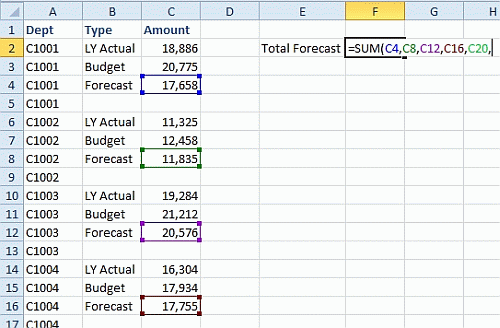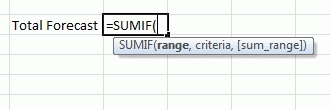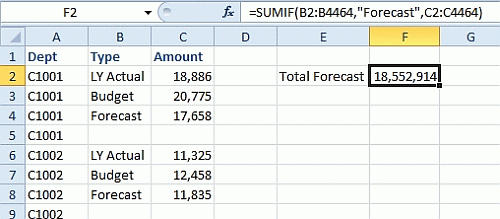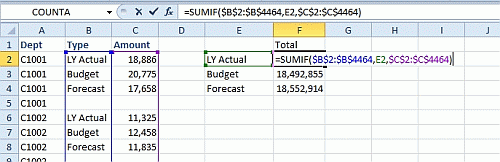Technology

# Automate the Selection and Sum of Variable Rows in Excel

Make quick work of summing variable rows choosing only data you specify based on row numbers and labels.

Editor’s Note: If you would like Bill Jelen to answer your Excel question in an upcoming column, send an e-mail note to [email protected] If he chooses your question as a topic for his column, you’ll win a book from CFO and MrExcel.com.

Kathleen W. wins a copy of Excel 2007 Miracles Made Easy for her question, “How can you set up a function to sum variable rows? For example, how would you sum every third cell where the row label is ‘forecast’?  Selecting each individual cell is too time-consuming if you have thousands of rows.”

Yes, building the formula by referring to each individual cell would take too long. Here’s my suggestion. First, take a look at the data set in Figure 1. It would take hours to finish the formula I’ve started there.

Fig. 1Formulas like =C4+C8+C12+C16+C20+ or =SUM(C4,C8,C12,C16,C20, have been around since the dawn of the spreadsheet in 1981. What you need to solve this problem is a function introduced in 1997, called SUMIF.

Fig. 2As you can see in Figure 2 (above), the function usually has three arguments. First, you specify a range of row labels. The second argument is a single value that you hope some row labels will match. The third argument is a range of numbers that correspond to the row labels in argument 1. Whenever the row label in the range matches the criteria, the corresponding value from the sum_range is included in the total.

One way to build the formula is to specify the criteria in quotes (see Figure 3):  =SUMIF(B2:B4464,”Forecast”,C2:C4464)

Fig. 3Or, you can enter the criteria in a cell and point to a cell. In Figure 4 (below), I’ve added dollar signs to make the first and third arguments absolute references, and copied the formula to build a small summary table to add up each of the three types of values in the data set.

Fig. 4Variations on SUMIF
Back in Figure 2, Microsoft shows the [sum_range] argument in square brackets, meaning that it is an optional argument. How could this be optional? If the range and sum_range are the same set of cells, you can leave off the sum_range. This would only happen if you were looking for numeric criteria, such as summing all cells above 20000:

=SUMIF(C2:C4464,”>20000”)

Invariably, once you master SUMIF, you will run into a problem where you need to sum based on two conditions. SUMIF cannot handle this. If you are using Excel 2007 or newer, consult Excel help for the new SUMIFS (plural) function. Before Excel 2007, you had to use the extremely complicated SUMPRODUCT function to solve the problem.

Bill Jelen is the author of 33 books about Microsoft Excel and the host of MrExcel.com. You can win one of those 33 books if your question is used for a future column. E-mail your question using the link in the byline of this article, or directly to [email protected].